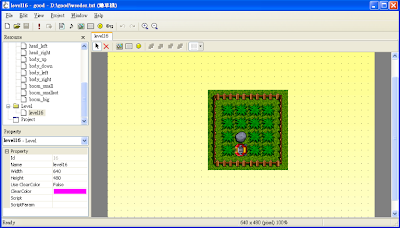2009年4月10日 星期五

實作鋤草機GamePlay

good的GamePlay使用Lua實作，所以繼續閱讀以下內容之前，我會先假設你已俱備使用Lua的基本能力，當然這也表示你對什麼是程式設計有基本的概念。good的物件類別是以Lua的Table實作的。下面的範例使用Lua的語法建立一個空的類別，也就是一個空的Table。雖然沒什麼功能，不過這樣子就能使用了，可以把它填入Script欄位。
Level = {}

Level.OnStep = function(param)
end

OnStep這個函式有個叫作param的參數。這個param參數傳來的就是前面提到過的物件的Instance。

if (Input.IsKeyPressed(Input.KEYS_LEFT)) then
end
Input.KEYS_LEFT是一個按鍵代碼，表示我們要檢查的是Left方向鍵的狀態。除了KEYS_LEFT外，還有KEYS_RIGHT、KEYS_DOWN、KEYS_UP、KEYS_RETURN等等。

local idSpr = Good.GetSpriteId(34)local x,y = Good.GetPos(idObj)

Good.SetPos(idObj, newx, newy)

local running = false
local body, head = 34, 35
local body_up, body_down, body_left, body_right = 9, 10, 11, 12

Level = {}

Level.OnStep = function(param)
if (nil == param.init) then
param.init = true
end

if (not running) then
if (Input.IsKeyPushed(Input.KEYS_LEFT)) then
running = true
end
return
end

local spd = 0.5
local x,y = Good.GetPos(body)
local dir = Good.GetSpriteId(body)

if (body_left == dir) then
x = x - spd
elseif (body_right == dir) then
x = x + spd
elseif (body_up == dir) then
y = y - spd
elseif (body_down == dir) then
y = y + spd
end

Good.SetPos(body, x, y)
endlocal movement = 0

Level.OnStep = function(param)
...
local dir = Good.GetSpriteId(body)
if (Input.IsKeyPushed(Input.KEYS_LEFT)) then
if (body_right ~= dir) then
end
elseif (Input.IsKeyPushed(Input.KEYS_RIGHT)) then
if (body_left ~= dir) then
end
elseif (Input.IsKeyPushed(Input.KEYS_UP)) then
if (body_down ~= dir) then
end
elseif (Input.IsKeyPushed(Input.KEYS_DOWN)) then
if (body_up ~= dir) then
end
end

movement = movement + spd

if (32 == movement) then
movement = 0

Good.SetSpriteId(body, body_up)
Good.SetSpriteId(body, body_down)
Good.SetSpriteId(body, body_left)
Good.SetSpriteId(body, body_right)
end
end
end
HitTest & KillObj

local hit = Good.FindObj(x, y, grass)

Good.KillObj(idObj)

local grass = 4
local gameover = false

Level.OnStep = function(param)
...
if (32 == movement) then
Good.KillObj(Good.FindObj(x, y, grass))
...
elseif (1 == movement) then
x = x + 32
y = y + 32
end

local hit = Good.FindObj(x, y, grass)
if (0 >= hit) then
gameover = true
end
end
end

Good模組提供了一個GenObj的方法可以讓我們動態生成一個物件。
local idNew = Good.GenObj(idParent, idRes, script)

local tile = Resource.GetTileByPos(idMap, x,y)
idMap是個地圖資源的ID，而x和y是相對於地圖左上角的座標。如果座標是有效的話，這個方法會回傳那個位置的地圖Tile的值，否則回傳0表示無效。那我們要怎麼知道我們要檢查的地圖Tile的值是多少？很簡單，打開地圖編輯器，檢視狀態列最右邊的欄位在括號中的數值就是滑鼠所指地圖Tile的值。local rock, ground, boom_small, boom_smallest, boom_big = 59, 57, 13, 14, 15

Level.OnStep = function(param)
...
if (32 == movement) then
...
elseif (1 == movement) then
...
local hit = Good.FindObj(x, y, grass)
if (0 >= hit) then
local tile = Resource.GetTileByPos(3, x - 224, y - 144)
local spr = boom_small
if (tile == rock) then
spr = boom_big
elseif (ground == tile) then
spr = boom_smallest
end
Good.GenObj(body, spr)
gameover = true
end
end
end